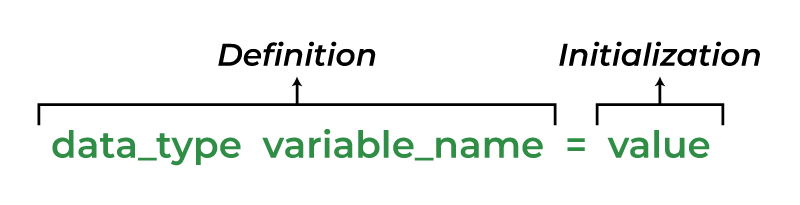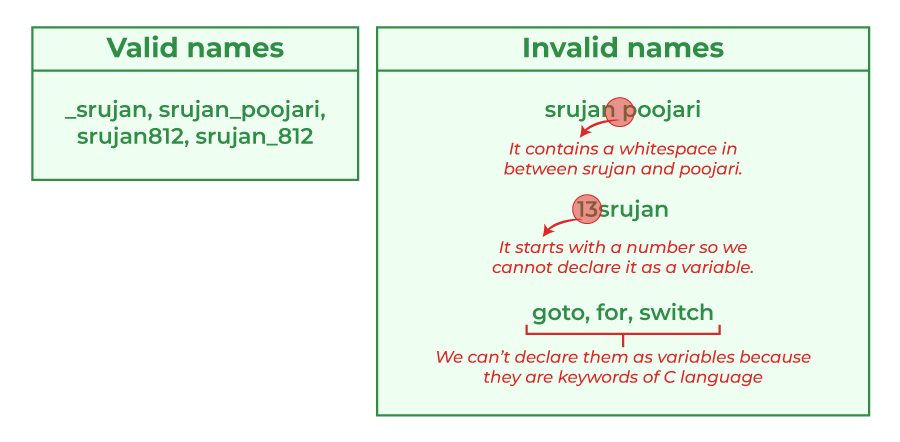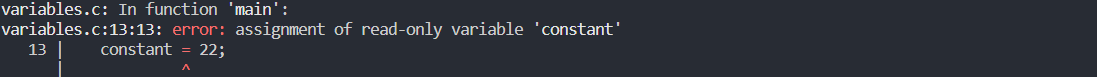Open in App
Not now

# Variables in C

• Difficulty Level : Easy
• Last Updated : 28 Dec, 2022

A variable in C is a memory location associated with some name in order to store some form of data and retrieve it when required. We can store different types of data in the variable and reuse the same variable for storing some other data any number of times.

For using a variable in C, we have to first define it to tell the compiler about its existence so that compiler can allocate the required memory to it.

Syntax:

```data_type variable_name = value;    // defining single variable
or
data_type variable_name1, variable_name2;    // defining multiple variable```
• data_type: Type of data that a variable can store.
• variable_name: Name of the variable given by the user.
• value: value assigned to the variable by the user.

There are 3 aspects of defining a variable:

#### Variable Declaration

Variable declaration in C tells the compiler about the existence of the variable with the given name and data type. No memory is allocated to a variable in the declaration.

#### Variable Definition

In the definition of a C variable, the compiler allocates some memory and some value to it. A defined variable will contain some random garbage value till it is not initialized.

#### Variable Initialization

Initialization of a variable is the process where the user assigns some meaningful value to the variable.Example:

## C

 `// C program to demonstrate the``// declaration, definition and``// initialization``#include ` `int` `main()``{``    ``// declaration with definition``    ``int` `defined_var;` `    ``printf``(``"Defined_var: %d\n"``, defined_var);` `    ``// initialization``    ``defined_var = 12;` `    ``// declaration + definition + initialization``    ``int` `ini_var = 25;` `    ``printf``(``"Value of defined_var after initialization: %d\n"``,defined_var);``    ``printf``(``"Value of ini_var: %d"``, ini_var);` `    ``return` `0;``}`

Output

```Defined_var: 0
Value of defined_var: 12
Value of ini_var: 25```

Note: In the above example, we have not demonstrated the variable declaration only as most of the modern C compilers declare and define the variable in single step. Although we can declare a variable in C by using extern keyword. To know more about variable declaration and definition, click here.

## Rules for naming variables

You can assign any name to the variable as long as it follows the following rules:

1. A variable name must only contain alphabets, digits, and underscore.
3. No whitespace is allowed within the variable name.
4. A variable name must not be any reserved word or keyword.## Types of Variables in C

We can classify variables on the basis of their scope, memory location, lifetime, etc. Following are the most common classification of the types of variables in C language.

### 1. Classification on the Basis of Scope

The scope of a variable is the region in which the variable exists it is valid to perform operations on it. Beyond the scope of the variable, we cannot access it and it is said to be out of scope.

On the basis of scope, C variables can be classified into two types:

1. Local Variables
2. Global Variables

#### A. Local Variable

Local variables in C are those variables that are declared inside a function or a block of code. Their scope is limited to the block or function in which they are declared.

## C

 `// C program to declare and print local variable inside a``// function.``#include ` `void` `function()``{``    ``int` `x = 10; ``// local variable``    ``printf``(``"%d"``, x);``}` `int` `main() { function(); }`

Output

`10`

In the above code, x can be used only in the scope of function(). Using it in the main function will give an error.

#### B. Global Variable

Global variables in C are those variables that are declared outside the function or a block of code. Their scope is the whole program i.e. we can access the global variable anywhere in the C program after it is declared.

Example:

## C

 `// C program to demonstrate use of global variable``#include ` `int` `x = 20; ``// global variable` `void` `function1() { ``printf``(``"Function 1: %d\n"``, x); }` `void` `function2() { ``printf``(``"Function 2: %d\n"``, x); }` `int` `main()``{` `    ``function1();``    ``function2();``    ``return` `0;``}`

Output

```Function 1: 20
Function 2: 20```

In the above code, both functions can use the global variable as global variables are accessible by all the functions.

Note: When we have same name for local and global variable, local variable will be given preference over the global variable by the compiler.

For accessing global variable in this case, we can use the method mention here.

### 2. Classification on the Basis of Storage Class

Storage Classes in C is the concept that helps us to determine the scope, lifetime, memory location, and default value (initial value) of a variable.

On the basis of storage classes, we can classify the C variables into 4 types:

1. Static Variables
2. Automatic Variables
3. Extern Variables
4. Register Variables

#### A. Static Variable

The static variables in C are those variables that are defined using the static keyword. They can be defined only once in a C program and their scope depends upon the region where they are declared (can be global or local).

The default value of static variables is zero.

As their lifetime is till the end of the program, they can retain their value for multiple function calls as shown in the example.

Syntax:

`static data_type variable_name = initial_value;`

Example:

## C

 `// C program to demonstrate use of static variable``#include ` `void` `function()``{``    ``int` `x = 20; ``// local variable``    ``static` `int` `y = 30; ``// static variable``    ``x = x + 10;``    ``y = y + 10;``    ``printf``(``"\tLocal: %d\n\tStatic: %d\n"``, x, y);``}` `int` `main()``{``    ``printf``(``"First Call\n"``);``    ``function();``    ``printf``(``"Second Call\n"``);``    ``function();``    ``printf``(``"Third Call\n"``);``    ``function();``    ``return` `0;``}`

Output

```First Call
Local: 30
Static: 40
Second Call
Local: 30
Static: 50
Third Call
Local: 30
Static: 60```

In the above example, we can see that the local variable will always print the same value whenever the function will be called whereas the static variable will print the incremented value in each function call.

#### B. Automatic Variable

All the local variables are automatic variables by default. They are also known as auto variables.

Their scope is local and their lifetime is till the end of the block. If we need, we can use the auto keyword to define the auto variables.

The default value of the auto variables is a garbage value.

Syntax:

```auto data_type variable_name;
or
data_type variable_name;    (in local scope)```

## C

 `// C program to demonstrate use of automatic variable``#include ` `void` `function()``{``    ``int` `x = 10; ``// local variable (also automatic)``    ``auto` `int` `y = 20; ``// automatic variable``    ``printf``(``"Auto Variable: %d"``, y);``}``int` `main()``{` `    ``function();``    ``return` `0;``}`

Output

`Auto Variable: 20`

In the above example, both x and y are automatic variables. The only difference is that variable y is explicitly declared with the auto keyword.

#### C. External Variables

External variables in C can be shared between multiple C files. We can declare an external variable using the extern keyword.

Their scope is global and they exist between multiple C files.

Syntax:

`extern data_type variable_name;`

Example:

```  myfile.h

extern int x=10;//external variable (also global)

program1.c
#include "myfile.h"
#include <stdio.h>
void printValue(){
printf("Global variable: %d", global_variable);
}```

In the above example, x is an external variable that is used in multiple C files.

#### D. Register Variables

Register variables in C are those variables that are stored in the CPU register instead of the conventional storage place like RAM. Their scope is local and exists till the end of the block or a function.

These variables are declared using the register keyword.

The default value of register variables is a garbage value.

Syntax:

`register data_type variable_name = initial_value;`

Example:

## C

 `// C program to demonstrate the definition of register``// variable``#include ` `int` `main()``{``    ``//    register variable``    ``register` `int` `var = 22;` `    ``printf``(``"Value of Register Variable: %d\n"``, var);``    ``return` `0;``}`

Output

`Value of Register Variable: 22`

NOTE: We cannot get the address of the register variable using addressof (&) operator because they are stored in the CPU register. The compiler will throw an error if we try to get the address of register variable.

## Constant Variable

Till now we have only seen the variables whose values can be modified any number of times. But C language also provides us a way to make the value of a variable immutable. We can do that by defining the variable as constant.

A constant variable in C is a read-only variable whose value cannot be modified once it is defined. We can declare a constant variable using the const keyword.

Syntax:

`const data_type variable_name = value;`

Note: We have to always initialize the const variable at the definition as we cannot modify its value after defining.

Example:

## C

 `// C Program to Demonstrate constant variable``#include ` `int` `main()``{``    ``// variable``    ``int` `not_constant;` `    ``// constant variable;``    ``const` `int` `constant = 20;` `    ``// changing values``    ``not_constant = 40;``    ``constant = 22;` `    ``return` `0;``}`

OutputMy Personal Notes arrow_drop_up